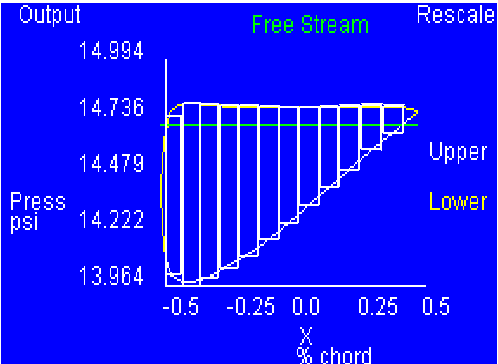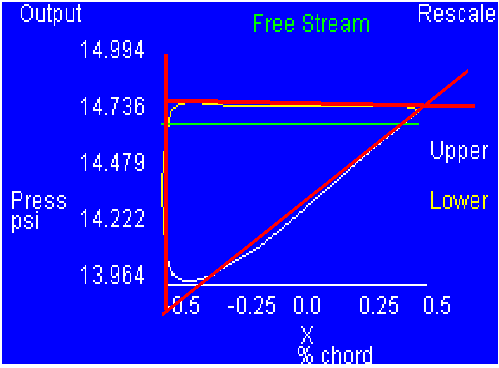PRINT THIS ANSWER SHEET TO FILL OUT AND TURN IN.

NAME_________________________________ CLASS____________________ DATE____________

## Getting a Little "Lift"out of Calculus A FoilSim Activity - Part I

Draw narrow rectangles with equal widths across the region bounded by the curves (as shown in Figure 2) and find the area of each. (Be careful with the scaling.) Create a table and record this information.Figure 2

Total Area (Lift):

Lift given in FoilSim:

Using the same graph, draw a straight line along the curves as shown in Figure 3.Find the slopes of each line and use them to find the respective equations.Use integration techniques to find the area of the enclosed region; this value represents the lift force. Compare your value to the value given by FoilSim and to your previous value. Figure 3

Total Area (Lift):

Lift given in FoilSim: